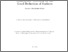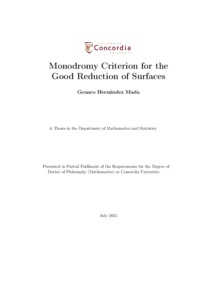Title:

# Monodromy Criterion for the Good Reduction of Surfaces

Hernandez Mada, Genaro (2015) Monodromy Criterion for the Good Reduction of Surfaces. PhD thesis, Concordia University.Preview
Text (application/pdf)
576kB

## Abstract

Let p>3 be a prime number and K a finite extension of Q_p. We consider a proper and smooth surface XK over K, with a semistable model X over the ring of integers OK of K. In this thesis, we give a criterion for the good reduction of XK for the case of K3 surfaces, in terms of the monodromy operator in the second De Rham cohomology group.
We don't use trascendental methods nor p-adic Hodge Theory as in other works concerning this problem. Instead, we first get a p-adic version of the Clemens-Schmid exact sequence and use it to study the degree of nilpotency of the monodromy operator N on the log-crystalline cohomology group of the special fiber Xs of the semistable model X.
By the work of Nakkajima, we can assume that Xs is a combinatorial K3 surface. Then, we prove that Xs is of type I iff N=0; Xs if of type II iff N is not zero and N^2=0; Xs is of type III iff N^2 is not zero. In particular, this implies that XK has good reduction if and only if the monodromy operator on its second De Rham cohomology is zero.
Finally, we also give some ideas on how to address the same problem for the case of Enriques surfaces. In particular, we prove that we are reduced to the case of K3 surfaces.

Divisions: Concordia University > Faculty of Arts and Science > Mathematics and Statistics Thesis (PhD) Hernandez Mada, Genaro Concordia University Ph. D. Mathematics 3 July 2015 Iovita, Adrian Monodromy, Good Reduction, K3 Surfaces 980454 GENARO HERNANDEZ MADA 28 Oct 2015 12:42 18 Jan 2018 17:51

## References:

\bibitem[AIK13]
FF. Andreatta, A. Iovita, M. Kim, {\it A $p$-adic non-abelian criterion for good reduction of curves}, (2013), available at www.mat.unimi.it/users/andreat/research.html
\bibitem[AM77]
MM. Artin, B. Mazur, {\it Formal groups arising from algebraic varieties}, Ann. Scient. \'Ecole Norm. Sup. 4e s\'erie, t. 10 (1977), 87-132.

\bibitem[Be97i]
PP. Berthelot, {\it Dualité de Poincaré et formule de Künneth en cohomologie rigide}, C. R. Acad. Sci. Paris, {\bf 325}, Série I (1997), pp. 493-498.

\bibitem[Be97ii]
PP. Berthelot, {\it Finitude et puret\'e cohomologique en cohomologie rigide}. Invent. Math. {\bf 128} (1997), 329-377.

\bibitem[BO78]
PP. Berthelot, A. Ogus, {\it Notes on Crystalline Cohomology}, Princeton University Press and University of Tokyo Press, 1978.

\bibitem[BGR]
SS. Bosch, U. G\"untzer, R. Remmert, {\it Non-Archimedean Analysis: a systematic approach to rigid analytic geometry}. Grundlehren der Mathematischen Wissenschaften, Vol. 261. Springer-Verlag (1984).

\bibitem[BPV]
WW. Barth, C. Peters, A. Ven, A. {\it Compact Complex Surfaces} Springer-Verlag (1984).

\bibitem[Br00]
CC. Breuil, {\it Groupes p-divisibles, groupes finis et modules filtr\'es}, Ann. of Math. (2) {\bf 152} (2000), no. 2, 489-549.
\bibitem[Ch99]
BB. Chiarellotto, {\it Rigid Cohomology and Invariant Cycles for a Semistable Log Scheme}. Duke Mathematical Journal. {\bf 97} (1999), no.1, 155-169.
\bibitem[Cl77]
CC.H. Clemens, {\it Degenerations of K\"ahler manifolds}. Duke Math J. {\bf 44} (1977), pp. 215-290.
\bibitem[CD]
FF. R. Cossec, I. V. Dolgachev, {\it Enriques Surfaces I}, Progress in Math. vol. 76, Birkhauser (1989).

\bibitem[Cr98]
RR. Crew, {\it Finiteness Theorems for the cohomology of an overconvergent isocrystal on a curve}. Ann. Sci. \'Ec. Norm. Sup\'er., {\bf 31}, (1998), 717-763.
\bibitem[CT03]
BB. Chiarellotto, N. Tsuzuki, {\it Cohomological descent of rigid cohomology for \'etale coverings}, Rend. Semin. Mat. Univ. Padova, {\bf 109}, (2003), 63-215.
\bibitem[CT12]
BB. Chiarellotto, N. Tsuzuki, {\it Clemens-Schmid exact sequence in characteristic $p$} arXiv:1111.0779 (2012)

\bibitem[CI99]
RR. Coleman, A. Iovita, {\it The Frobenius and monodromy operators for curves and abelian varieties}, Duke Mathematical Journal {\bf 97} (1999), no. 1, 171-215.

\bibitem[De81]
PP. Deligne, {\it Rel\evement des surfaces $K3$ en caract\'eristique nulle}. Surfaces alg\'ebriques, S\'eminaire de G\'eom\'etrie alg\'ebrique d'Orsay 1976-1978. Lecture Notes in Math. 868, Springer-Verlag. New York (1981), pp. 58-79.

\bibitem[El73]
RR. Elkik, {\it Solutions d'\'equations \a coefficients dans un anneau hens\'elien}, Annales scientifiques de l'ENS, 4\`eme s\'erie, t. 6 (1973), pp. 553-604.

\bibitem[FV]
JJ. Fresnel, M. Van der Put, {\it Rigid Analytic Geometry and its Applications}. Progress in Mathematics, Vol. 218. Birkh\"auser Boston Inc. (2004).

\bibitem[HK94]
OO. Hyodo, K. Kato, {\it Semi-stable Reduction and Crystalline Cohomology with Logarithmic Poles}, P\'eriode $p$-adiques (Bures sur Yvette 1988), Ast\'erisque, {\bf 223} (1994), 221-268.

\bibitem[It05]
TT. Ito, {\it Weight-monodromy conjecture over equal characteristic local fields}, American Journal of Mathematics, vol. 127, no. 3, (2005), pp. 647-658.

\bibitem[Ka96]
FF. Kato, {\it Log smooth deformation theory}. Tohoku Math. Jour. {\bf 48} (1996), 317-354.
\bibitem[Ka89]
KK. Kato, {\it Logarithmic structures of Fontaine-Illusie}. Algebraic analysis, geometry and number theory, the Johns Hopkins Univ. Press (1989), pp. 191-224.
\bibitem[Kw93]
YY. Kawamata, {\it Semistable Minimal Models of Threefolds in Positive or Mixed Characteristic.} arXiv:alg-geom/9303001(1993).

\bibitem[Kw98]
YY. Kawamata, {\it Index 1 covers of log terminal surface singularities}. arXiv:math/9802044 (1998).

\bibitem[Ku77]
VV. Kulikov, {\it Degenerations of $K3$ surfaces and Enriques' surfaces}. Math. USSR Izv. {\bf 11} (1977), 957-989.
\bibitem[La73]
AA. Landman, {\it On the Picard-Lefschetz transformations}. Trans. AMS {\bf 181} (1973), pp. 89-126.
\bibitem[Lz14]
CC. Lazda, {\it Rigid rational homotopy types}. arXiv:1306.6446 (2014).
\bibitem[Li13]
CC. Liedtke, {\it Algebraic surfaces in positive characteristic} in Birational Geometry, Rational Curves and Arithmetic. F. Bogomolov, B. Hasset, Y. Tschnkel Eds., Springer 2013. 229-292.
\bibitem[Liu]
QQ. Liu, {\it Algebraic Geometry and Arithmetic Curves}. Oxford Graduate Texts in Mathematics {\bf 6}. Oxford University Press 2002.
\bibitem[LS]
BB. Le Stum, {\it Rigid Cohomology}. Cambridge Tracts in Mathematics {\bf 172}. Cambridge University Press 2007.
\bibitem[LM14]
CC. Liedtke, Y. Matsumoro, {\it Good Reduction of $K3$ Surfaces}, arXiv:1411.4797 (2014).

\bibitem[LP80]
EE. Looijenga, C. Peters, {\it Torelli theorems for K\"ahler K3 surfaces.} Compositio Mathematica, {\bf 42} (1980), 145?186.
\bibitem[Ma14]
YY. Matsumoto, {\it Good reduction criterion for $K3$ surfaces}, arXiv:1401.1261 (2014).
\bibitem[Mau12]
DD. Maulik, {\it Supersingular K3 surfaces for large primes,} arXiv:1203.2889 (2012).
\bibitem[Mo81]
DD.R. Morrison, {\it Semistable Degenerations of Enriques and Hyperelliptic Surfaces}, Duke Math. J., Vol. 48, No.1 (1981), 197-249.
\bibitem[Mo84]
DD.R. Morrison, {\it The Clemens-Schmid exact sequence and applications,} Topics in Transcendental Algebraic Geometry, Annals of Mathematics Studies, vol. 106. Princeton University Press, Princeton (1984), pp. 101-119.
\bibitem[Mk93]
AA. Mokrane, {\it La suite spectrale des poids en cohomologie de Hyodo-Kato}. Duke Math. J. {\bf 72} (1993), 301-337.
\bibitem[Na00]
YY. Nakkajima, {\it Liftings of Simple Normal Crossing Log $K3$ and Log Enriques Surfaces in Mixed Characteristics,} Algebraic Geometry, {\bf 9} (2000), 355-393.

\bibitem[Ny83]
NN. O. Nygaard, {\it The Tate conjecture for ordinary $K3$ surfaces over finite fields}, Invent. Math. {\bf 74} (1983), 213-237.

\bibitem[Oda95]
TT. Oda, {\it A note on ramification of the Galois representation on the fundamental group of an algebraic curve. II}. J. Number Theory {\bf 53} (1995), no. 2, 342-355.
\bibitem[Pe14]
JJ. R. P\'erez Buend\'ia, {\it A Crystalline Criterion for Good Reduction on Semi-stable $K3$-Surfaces over a $p$-Adic Field} (2014), available at http://spectrum.library.concordia.ca/978195/

\bibitem[Po]
DD. Popescu, {\it General N\'eron Desingularization and Approximation}. Nagoya Math. J. {\bf 104} (1986), 85-115.

\bibitem[PP81]
UU. Persson, H. Pinkham, {\it Degeneration of Surfaces with Trivial Canonical Bundle}. Annals of Mathematics, Second series, {\bf 113}, No.1, 45-66.

\bibitem[Sc68]
MM. Schlessinger, {\it Functors of Artin Rings}. Trans. AMS {\bf 30} (1968), 208-222.

\bibitem[Siu]
YY. T. Siu, {\it Every K3 surface is K\"ahler}, Invent. Math. {\bf 73} (1983), no. 1, 139-150.
\bibitem[ST68]
JJ.P. Serre, J. Tate, {\it Good reduction of abelian varieties}. Ann. of Math. (2) {\bf 88} (1968), 492-517.

\bibitem[Sh08]
AA. Shiho, {\it Relative Log Convergent Cohomology and Relative Rigid Cohomology I} arXiv:0707.1742 (2008).

\bibitem[St76]
JJ. Steenbrink, {\it Limits of Hodge Structures}, Inventiones mathematicae {\bf 31} (1976), no.3, 229-257.
\bibitem[Sw95]
RR. Swan,
N\'eron-Popescu desingularization. {\it Algebra and geometry} (Taipei 1995), Lect. Algebra Geom. 2 Int. Press, Cambridge, MA (1998), pp. 135-192.

\bibitem[Ts99]
TT. Tsuji, {\it $p$-adic \'etale cohomology and crystalline cohomology in the semi-stable reduction case}. Invent. Math. 137 (1999), {\bf 1}, 233-411.
All items in Spectrum are protected by copyright, with all rights reserved. The use of items is governed by Spectrum's terms of access.

Repository Staff Only: item control pageResearch related to the current document (at the CORE website)
Back to top